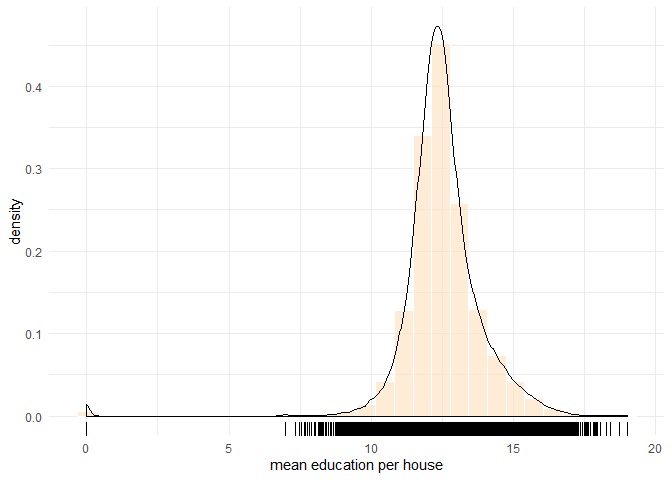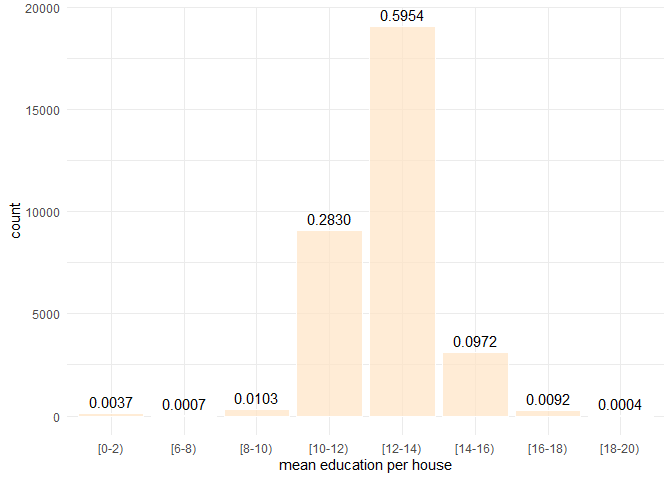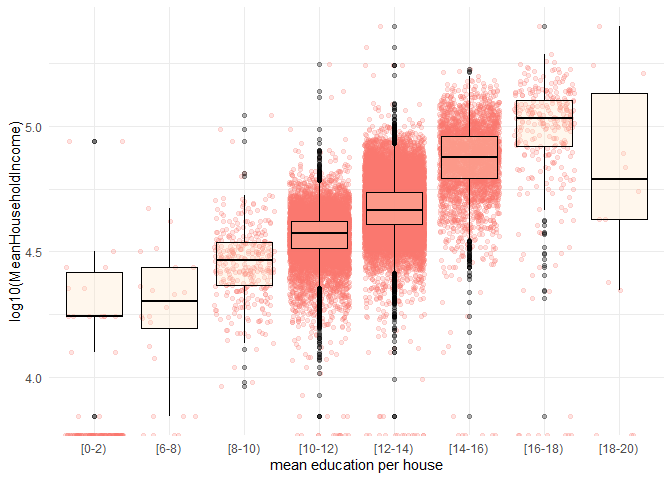# Data Binning and Plotting in R

Updated on 9/28/2019

Data binning is a basic skill that a knowledge worker or data scientist must have. When we want to study patterns collectively rather than individually, individual values need to be categorized into a number of groups beforehand. We can group values by a range of values, by percentiles and by data clustering.

Grouping by a range of values is referred to as data binning or bucketing in data science, i.e., categorizing a number of continuous values into a smaller number of bins (buckets). Each bucket defines an interval. A category name is assigned each bucket. An example of this is the grading system in the U.S. where a $90%$ grade or better is an A, 80–89% is B, etc. The intervals can be set to either equal-width or varying-width. For instance, the 5-point Likert data can be converted into categories with 4 and 5 being “High”, 3 being “Medium”, and 1 and 2 being “Low”.

This post shows two examples of data binning in R and plot the bins in a bar chart as well. The first one uses R Base function cut. The second one uses the data manipulation functions in the dplyr package.

# The cut function: Categorizing Continuous Values into Groups

The example is categorizing mean education level per house which was originally measured by numeric values ranged from 0 until 19, perform data binning to place each value into one bucket if the value falls into the interval that the bucket covers.

## Data

The dataset contains 32038 observations for mean education level per house. Load the data into R.

library(tidyverse)
data <- read_delim(file = "../data/zipIncome.csv", delim = ',')
v <- data %>% select(MeanEducation,MeanHouseholdIncome) #pick the variable 

Run summary with v to find the range of mean education:

summary(v)
##  MeanEducation   MeanHouseholdIncome
##  Min.   : 0.00   Min.   :     0
##  1st Qu.:11.88   1st Qu.: 37644
##  Median :12.43   Median : 44163
##  Mean   :12.52   Mean   : 48245
##  3rd Qu.:13.10   3rd Qu.: 54373
##  Max.   :19.00   Max.   :250000

The summary shows the range, $$[0,19]$$. The median education level per house is $$12.43$$, to the left of, but very close to the mean. The frequency chart with a density curve shows a bell-shaped distribution with a dense area between 10 and 16.

ggplot(data = v, mapping = aes(x=MeanEducation)) +
geom_histogram(aes(y=..density..),fill="bisque",color="white",alpha=0.7) +
geom_density() +
geom_rug() +
labs(x='mean education per house') +
theme_minimal()data binning plot

While the density curve is informative, it can be too technical for average users to read. In this case, a better way is binning the values into discrete categories and plotting the count of each bin in bars.

## Defining breaks and cut a vector into bins

# set up cut-off values
breaks <- c(0,2,4,6,8,10,12,14,16,18,20)
# specify interval/bin labels
tags <- c("[0-2)","[2-4)", "[4-6)", "[6-8)", "[8-10)", "[10-12)","[12-14)", "[14-16)","[16-18)", "[18-20)")
# bucketing values into bins
group_tags <- cut(v$MeanEducation, breaks=breaks, include.lowest=TRUE, right=FALSE, labels=tags) # inspect bins summary(group_tags) ## [0-2) [2-4) [4-6) [6-8) [8-10) [10-12) [12-14) [14-16) [16-18) ## 120 0 0 24 330 9067 19075 3115 294 ## [18-20) ## 13 The code chunk above produces a factor group_tags which maps each original education value into one of the eleven bins. Each level is named by a string in the vector labels. Each value in bins indicates the interval a value falls into. The summary of group_tags lists the count in each bin. cut by default outputs an unordered factor. To get an ordered factor, rebuild the factor from group_tags: education_groups <- factor(group_tags, levels = labels, ordered = TRUE) ## Bar Chart Plot the bins: ggplot(data = as_tibble(group_tags), mapping = aes(x=value)) + geom_bar(fill="bisque",color="white",alpha=0.7) + stat_count(geom="text", aes(label=sprintf("%.4f",..count../length(group_tags))), vjust=-0.5) + labs(x='mean education per house') + theme_minimal()data binning plot # Categorizing by the dplyr Functions ## Define bucket intervals and give names tags <- c("[0-2)","[2-4)", "[4-6)", "[6-8)", "[8-10)", "[10-12)","[12-14)", "[14-16)","[16-18)", "[18-20)") ## Place mean education levels into buckets. Store groups as a new column. v <- data %>% select(MeanEducation,MeanHouseholdIncome) #pick the variable vgroup <- as_tibble(v) %>% mutate(tag = case_when( MeanEducation < 2 ~ tags, MeanEducation >= 2 & MeanEducation < 4 ~ tags, MeanEducation >= 4 & MeanEducation < 6 ~ tags, MeanEducation >= 6 & MeanEducation < 8 ~ tags, MeanEducation >= 8 & MeanEducation < 10 ~ tags, MeanEducation >= 10 & MeanEducation < 12 ~ tags, MeanEducation >= 12 & MeanEducation < 14 ~ tags, MeanEducation >= 14 & MeanEducation < 16 ~ tags, MeanEducation >= 16 & MeanEducation < 18 ~ tags, MeanEducation >= 18 & MeanEducation < 20 ~ tags )) summary(vgroup) ## MeanEducation MeanHouseholdIncome tag ## Min. : 0.00 Min. : 0 Length:32038 ## 1st Qu.:11.88 1st Qu.: 37644 Class :character ## Median :12.43 Median : 44163 Mode :character ## Mean :12.52 Mean : 48245 ## 3rd Qu.:13.10 3rd Qu.: 54373 ## Max. :19.00 Max. :250000 The new column tag from case_when is a character vector. To make it a factor, do; vgroup$tag <- factor(vgroup$tag, levels = tags, ordered = FALSE) summary(vgroup$tag)
##   [0-2)   [2-4)   [4-6)   [6-8)  [8-10) [10-12) [12-14) [14-16) [16-18)
##     120       0       0      24     330    9067   19075    3115     294
## [18-20)
##      13

# BoxPlot: Distribution of Mean Household Income for Each Level of Education

ggplot(data = vgroup, mapping = aes(x=tag,y=log10(MeanHouseholdIncome))) +
geom_jitter(aes(color='blue'),alpha=0.2) +
geom_boxplot(fill="bisque",color="black",alpha=0.3) +
labs(x='mean education per house') +
guides(color=FALSE) +
theme_minimal()data binning plot

Set up a Python environment for doing Data Science in Jupyter Notebook with Conda virtual environment